Note: This is an archvied version of our old webpage. Some links might be broken. The current one can be found here.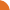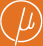Chair for Foundations of Software Reliability and Theoretical Computer Science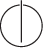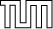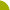Publications - Computing Least Fixed Points of Probabilistic Systems of PolynomialsReference: Javier Esparza, Andreas Gaiser, and Stefan Kiefer. Computing least fixed points of probabilistic systems of polynomials. Technical report, Technische Universität München, Institut für Informatik, December 2009. Abstract: We study systems of equations of the form X1 = f1(X1, ..., Xn), ..., Xn = fn(X1, ..., Xn), where each fi is a polynomial with nonnegative coefficients that add up to 1. The least nonnegative solution, say mu, of such equation systems is central to problems from various areas, like physics, biology, computational linguistics and probabilistic program verification. We give a simple and strongly polynomial algorithm to decide whether mu=(1, ..., 1) holds. Furthermore, we present an algorithm that computes reliable sequences of lower and upper bounds on mu, converging linearly to mu. Our algorithm has these features despite using inexact arithmetic for efficiency. We report on experiments that show the performance of our algorithms. Suggested BibTeX entry: @techreport{EGK10:stacsTechRep,     author = {Javier Esparza and Andreas Gaiser and Stefan Kiefer},     institution = {Technische Universit{\"a}t M{\"u}nchen, Institut f\"{u}r Informatik},     month = {December},     title = {Computing Least Fixed Points of Probabilistic Systems of Polynomials},     year = {2009} }